## Depth First Search-

• Depth First Search or DFS is a graph traversal algorithm.
• It is used for traversing or searching a graph in a systematic fashion.
• DFS uses a strategy that searches “deeper” in the graph whenever possible.
• Stack data structure is used in the implementation of depth first search.

## DFS Example-

Consider the following graph-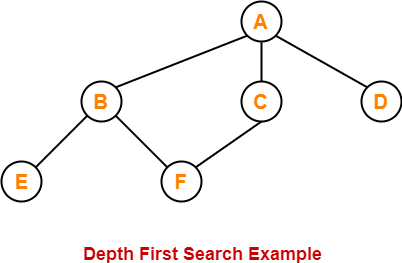The depth first search traversal order of the above graph is-

A, B, E, F, C, D

## Depth First Search Algorithm-

#### DFS (V,E)

for each vertex u in V[G]

do color[v] ← WHITE

π[v] ← NIL

time ← 0

for each vertex v in V[G]

do if color[v] ← WHITE

then Depth_First_Search(v)

### Depth_First_Search (v)

color[v] ← GRAY

time ← time + 1

d[v] ← time

for each vertex u adjacent to v

do if color[u] ← WHITE

π[u] ← v

Depth_First_Search(u)

color[v] ← BLACK

time ← time + 1

f[v] ← time

## Explanation-

The above depth first search algorithm is explained in the following steps-

## Step-01

Create and maintain 4 variables for each vertex of the graph.

For any vertex ‘v’ of the graph, these 4 variables are-

### 1. color[v]-

• This variable represents the color of the vertex ‘v’ at the given point of time.
• The possible values of this variable are- WHITE, GREY and BLACK.
• WHITE color of the vertex signifies that it has not been discovered yet.
• GREY color of the vertex signifies that it has been discovered and it is being processed.
• BLACK color of the vertex signifies that it has been completely processed.

### 2. Π[v]-

This variable represents the predecessor of vertex ‘v’.

### 3. d[v]-

This variable represents a timestamp when a vertex ‘v’ is discovered.

### 3. f[v]-

This variable represents a timestamp when the processing of vertex ‘v’ is completed.

## Step-02

For each vertex of the graph, initialize the variables as-

• color[v] = WHITE
• π[v] = NIL
• time = 0 (Global Variable acting as a timer)

### Step-03

Repeat the following procedure until all the vertices of the graph become BLACK-

Consider any white vertex ‘v’ and call the following Depth_First_Search function on it.

#### Depth_First_Search (G,v)

1. color[v] = GRAY

2. time = time + 1

3. d[v] = time

4. For each adjacent WHITE vertex ‘u’ of ‘v’, set π[u] = v and call Depth_First_Search (G,u)

5. color[v] = BLACK

6. time = time + 1

7. f[v] = time

## DFS Time Complexity-

The total running time for Depth First Search is θ (V+E).

## Types of Edges in DFS-

After a DFS traversal of any graph G, all its edges can be put in one of the following 4 classes-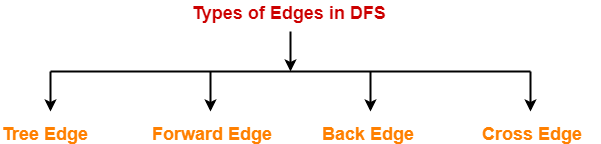1. Tree Edge
2. Back Edge
3. Forward Edge
4. Cross Edge

## 1. Tree Edge-

• A tree edge is an edge that is included in the DFS tree.

## 2. Back Edge-

 An edge from a vertex ‘u’ to one of its ancestors ‘v’ is called as a back edge. A self-loop is considered as a back edge.

A back edge is discovered when-

• DFS tries to extend the visit from a vertex ‘u’ to vertex ‘v’
• And vertex ‘v’ is found to be an ancestor of vertex ‘u’ and grey at that time.

### 3. Forward Edge-

 An edge from a vertex ‘u’ to one of its descendants ‘v’ is called as a forward edge.

A forward edge is discovered when-

• DFS tries to extend the visit from a vertex ‘u’ to a vertex ‘v’
• And finds that color(v) = BLACK and d(v) > d(u).

### 4. Cross Edge-

 An edge from a vertex ‘u’ to a vertex ‘v’ that is neither its ancestor nor its descendant is called as a cross edge.

A cross edge is discovered when-

• DFS tries to extend the visit from a vertex ‘u’ to a vertex ‘v’
• And finds that color(v) = BLACK and d(v) < d(u).

## Problem-

Compute the DFS tree for the graph given below-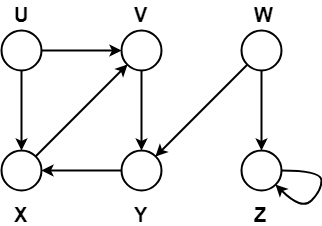Also, show the discovery and finishing time for each vertex and classify the edges.

## Solution-

Initially for all the vertices of the graph, we set the variables as-

• color[v] = WHITE
• π[v] = NIL
• time = 0 (Global)

Let us start processing the graph from vertex U.

### Step-01:

• color[U] = GREY
• time = 0 + 1 = 1
• d[U] = 1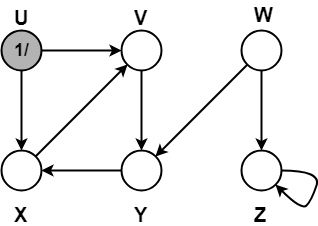### Step-02:

• π[V] = U
• color[V] = GREY
• time = 1 + 1 = 2
• d[V] = 2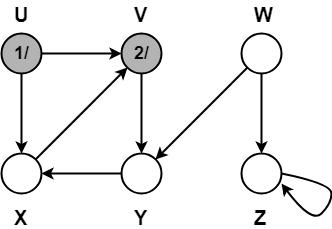### Step-03:

• π[Y] = V
• color[Y] = GREY
• time = 2 + 1 = 3
• d[Y] = 3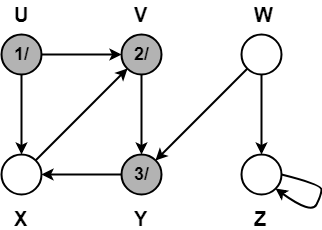### Step-04:

• π[X] = Y
• color[X] = GREY
• time = 3 + 1 = 4
• d[X] = 4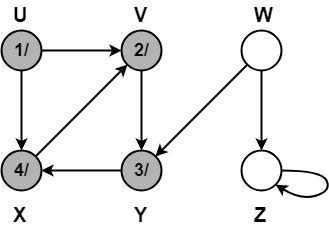### Step-05:

When DFS tries to extend the visit from vertex X to vertex V, it finds-

• Vertex V is an ancestor of vertex X since it has already been discovered
• Vertex V is GREY in color.

Thus, edge XV is a back edge.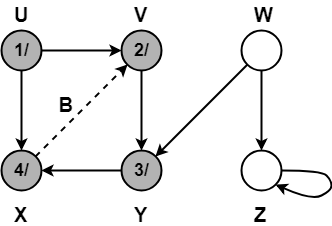### Step-06:

• color[X] = BLACK
• time = 4 + 1 = 5
• f[X] = 5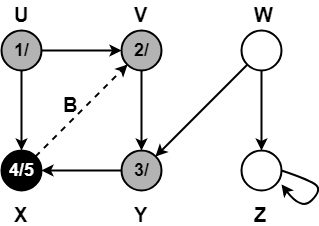### Step-07:

• color[Y] = BLACK
• time = 5 + 1 = 6
• f[Y] = 6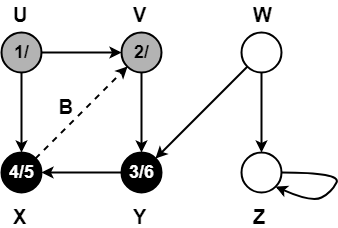### Step-08:

• color[V] = BLACK
• time = 6 + 1 = 7
• f[V] = 7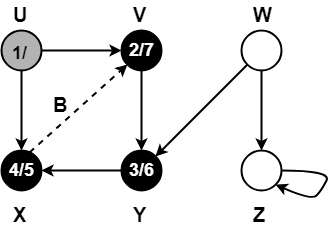### Step-09:

When DFS tries to extend the visit from vertex U to vertex X, it finds-

• Vertex X has already been completely processed i.e. vertex X has finished and is black.
• But vertex U has still not finished.

Alternatively,

When DFS tries to extend the visit from vertex U to vertex X, it finds-

• Color(X) = BLACK
• d(X) > d(U)

Thus, edge UX is a forward edge.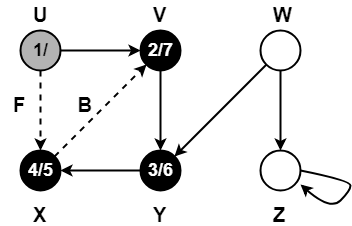### Step-10:

• color[U] = BLACK
• time = 7 + 1 = 8
• f[U] = 8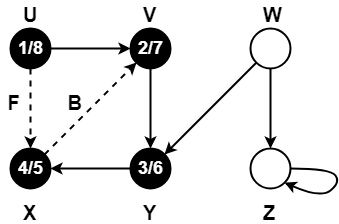### Step-11:

• color[W] = GREY
• time = 8 + 1 = 9
• d[W] = 9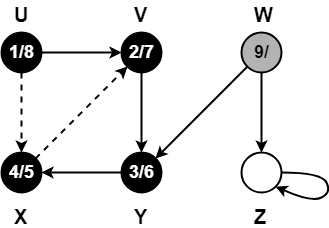### Step-12:

When DFS tries to extend the visit from vertex W to vertex Y, it finds-

• Vertex Y has already been completely processed i.e. vertex Y has finished.
• Vertex Y is neither a descendant nor an ancestor of vertex W.

Alternatively,

When DFS tries to extend the visit from vertex W to vertex Y, it finds-

• Color(Y) = BLACK
• d(Y) < d(W)

Thus, edge WY is a cross edge.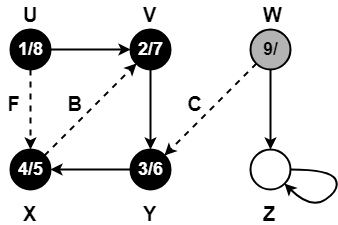### Step-13:

• π[Z] = W
• color[W] = GREY
• time = 9 + 1 = 10
• d[W] = 10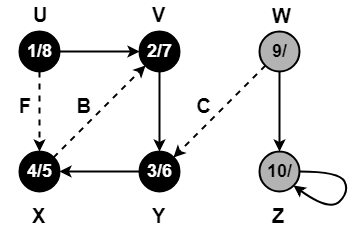### Step-14:

Since, self-loops are considered as back edges.

Therefore, self-loop present on vertex Z is considered as a back edge.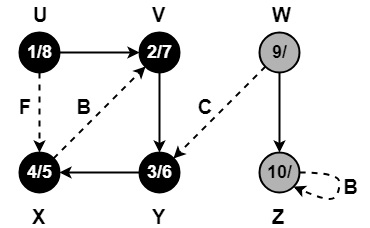### Step-15:

• color[Z] = BLACK
• time = 10 + 1 = 11
• f[Z] = 11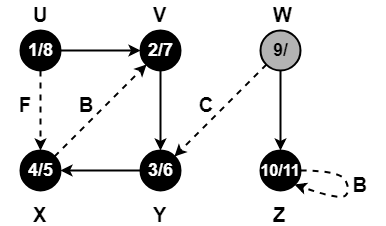### Step-16:

• color[W] = BLACK
• time = 11 + 1 = 12
• f[W] = 12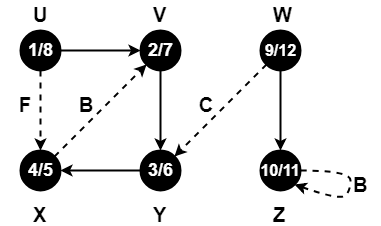Since all the vertices have turned black, so we stop.

This is how a given graph is traversed using Depth First Search (DFS) technique.

To gain better understanding about Depth First Search Algorithm,

Watch this Video Lecture